IMO Shortlist 2013 problem C7

Kvaliteta:
Avg: 0,0
Težina:
Avg: 9,0
Let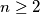$n \geq 2$ be an integer. Consider all circular arrangements of the numbers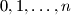$0, 1, \ldots, n$; the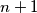$n + 1$ rotations of an arrangement are considered to be equal. A circular arrangement is called beautiful if, for any four distinct numbers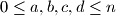$0 \leq a, b, c, d \leq n$ with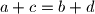$a + c = b + d$, the chord joining numbers$a$ and$c$ does not intersect the chord joining numbers$b$ and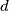$d$.

Let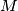$M$ be the number of beautiful arrangements of$0, 1, \ldots, n$. Let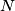$N$ be the number of pairs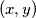$(x, y)$ of positive integers such that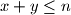$x + y \leq n$ and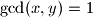$\operatorname{gcd}(x, y) = 1$. Prove that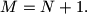Izvor: Russia